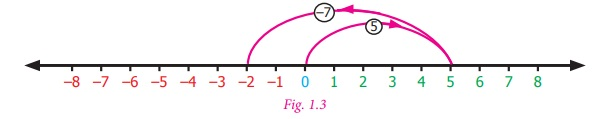Home | | Maths 7th Std | Number System

# Number System

Learning objectives ● To understand the concept of addition and subtraction of integers. ● To understand the concept of multiplication and division of integers. ● To understand the properties of four fundamental operations applied to integers. ● To solve applied problems using the four fundamental operations on integers.

Chapter 1

NUMBER SYSTEMLearning objectives

● To understand the concept of addition and subtraction of integers.

● To understand the concept of multiplication and division of integers.

● To understand the properties of four fundamental operations applied to integers.

● To solve applied problems using the four fundamental operations on integers.

Recap

Integers are a collection of natural numbers, zero and negative numbers.

We denote this collection by .

Negative integers are represented on the number line to the left of zero and positive integers to the right of zero.Every integer on this number line is placed in an increasing order from left to right.

The integers at each point A, B, C, D in the figure given below are A = +3, B = +7, = −1 and C = −5 .Try these

1. Write the following integers in ascending order: –5, 0, 2, 4, –6, 10, –10

Answer: –10, –6, –5, 0, 2, 4, 10

2. If the integers –15, 12, –17, 5, –1, –5, 6 are marked on the number line then the integer on the extreme left is –17.3. Complete the following pattern:

50, ___, 30, 20, ____, 0, −10 , ___, ___, −40 , ___, ___.

50, 40, 30, 20, 10, 0, –10 , –20–30, –40 , –50–60.

4. Compare the given numbers and write “<”, “>” or “=” in the boxes.

(a) – 65 ___ 65 (b) 0 ___ 1000 (c) −2018 ___  −2018

(a) –65 65 (b) 0 < 1000 (c) –2018  = – 2018

5. Write the given integers in descending order :

–27, 19, 0, 12, –4, –22, 47, 3, –9, –35

Answer: 47, 19, 12, 3, 0, –4, –9, –22, –27, –35

Introduction

In class VI, we studied how to compare and arrange integers. Now, let us try to add and subtract integers.

We know 7–5 = 2. But, what is 5–7? Let us try to take away 7 from 5 using the number line. As the integers on the number line increases from left to right, we should move to the left of zero to do this subtraction.Is it really necessary to do this? Have you come across any such situations in life? Yes, there are situations like increase or decrease in temperature, amount deposited and withdrawn from an account, profit and loss in a business are all instances where integers are involved.

MATHEMATICS ALIVE – NUMBER SYSTEM IN REAL LIFETags : Term 1 Chapter 1 | 7th Maths , 7th Maths : Term 1 Unit 1 : Number System
Study Material, Lecturing Notes, Assignment, Reference, Wiki description explanation, brief detail
7th Maths : Term 1 Unit 1 : Number System : Number System | Term 1 Chapter 1 | 7th Maths